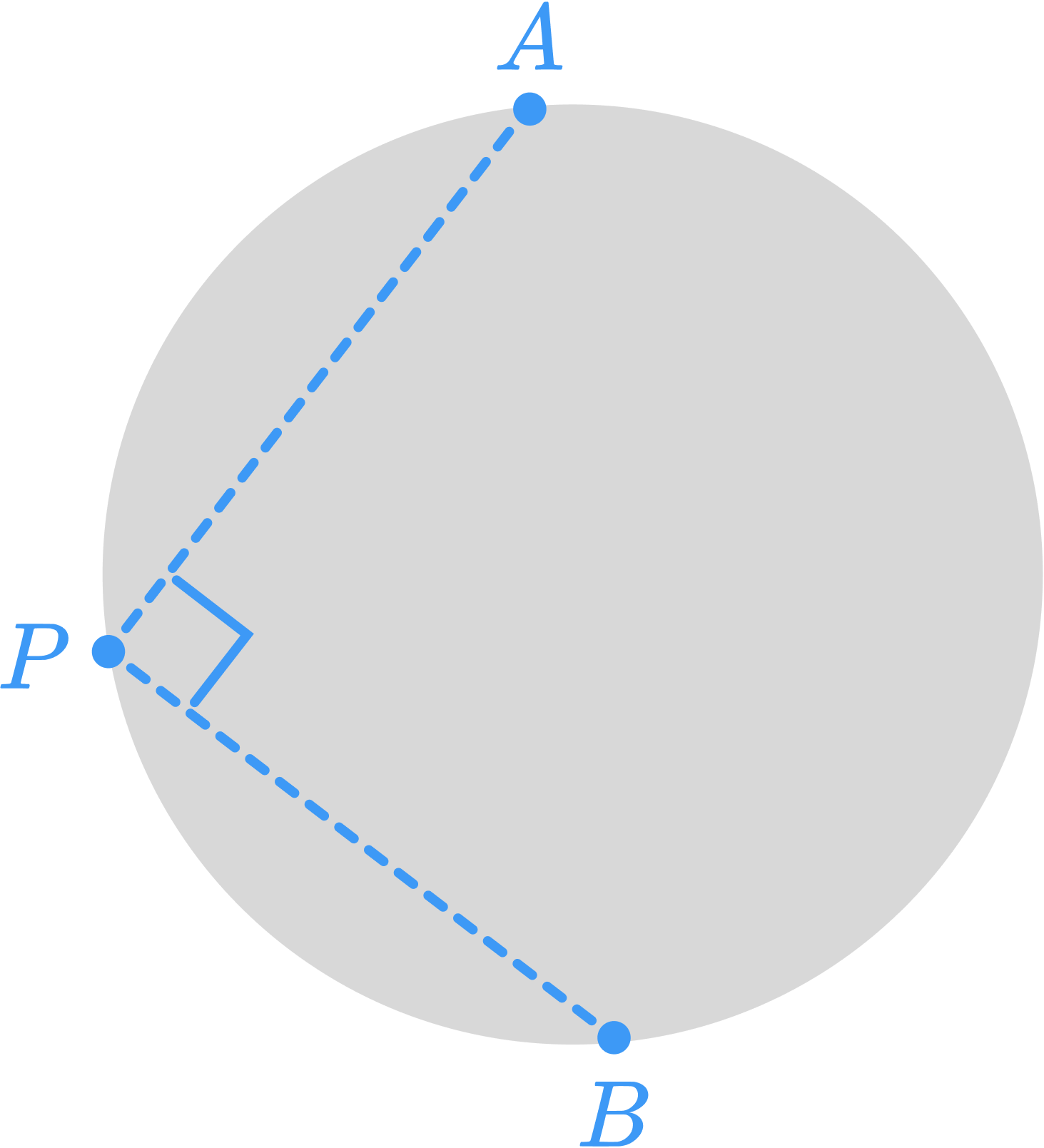# Perpendicular Is The Key

Geometry Level 1Let $P, A$ and $B$ be three distinct points on the circumference of a circle with radius of 5 units. The segments $PA$ and $PB$ are perpendiculars. What is the value of $PA^2 + PB^2 ?$

×